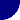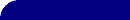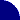# The Inductance of Your Electrode!RESOURCES > EIS > INDUCTANCE > CALCULATOR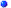Even a single, straight piece of wire has some inductance!We generally associate inductance with a loop or coil of wire. However, even a straight piece of wire, or your electrode, has some self-inductance. This can be important if you are dealing with low impedances (< 1 ohm) at high frequencies (> 10kHz).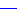My good, old 1962-63 edition of the CRC Handbook is a wealth of information! It contains a section on "Radio Formulae" (ref 1) that gives the inductance of a straight piece of round wire or rod:In this equation, L is the inductance in nH (10-9 henry), l is the length and d is the diameter of the wire/rod (both in cm). µ is the permeability of the material (=1.0, except for iron and other ferromagnetic materials). Home What's New? ElectrochemistryResourcesCONTENTS The BookstoreCONTENTS Search Tell Us !How useful wasthis information?

 Wire Diameter (d)  = m cm mm in Wire Length (l) = m cm mm in Permeability (µ) = (1.0 EXCEPT for IRON) Inductance = nH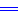Uses JavaScript. You must have a JavaScript capable browser, and have scripting enabled.Download the newest Internet Explorer or Firefox now !

The calculator, above, actually uses a slightly more complicated form of the equation (ref 2). The differences are small, even for fairly "squat" electrodes with d / l of 0.2 (x~1.005).Note: This equation applies to an isolated round wire far from a ground plane or earth. For other geometries, see references 2 or 4. It also applies to frequencies that are accessible to the electrochemist, generally <1MHz.More About ...
 Instrumental artifacts Inductance at Low Frequency Inductance at High Frequency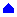REFERENCES(1) "Handbook of Chemistry and Physics, 44th Ed.", Chemical Rubber Publishing Co., Cleveland, OH, 1962. Yes, I got it NEW.(2) "Inductance Calculations", F. W. Grover, Dover Publications, 2004 .(3) http://www.ee.scu.edu/eefac/healy/indwire.html(4) http://home.san.rr.com/bushnell/self_inductance.htm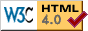© Copyright 2004-2014 Research Solutions & Resources LLC